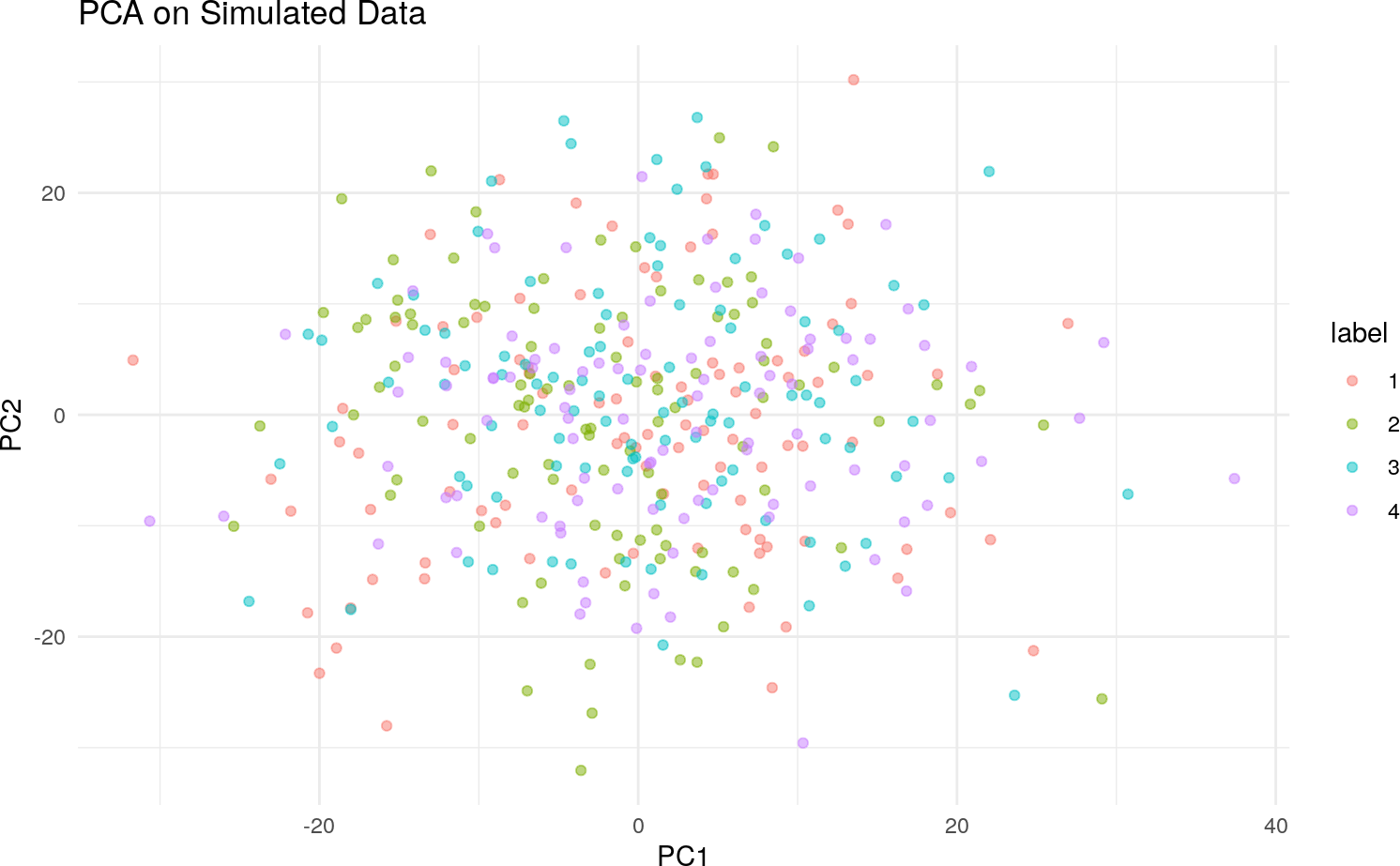# 1 Introduction

Data pre-processing and exploratory data analysis and are two important steps in the data science life-cycle. As datasets become larger and the signal weaker, their importance increases. Thus, methods that are capable of extracting the signal from such datasets is badly needed. Often, these steps rely on dimensionality reduction techniques to isolate pertinent information in data. However, many of the most commonly-used methods fail to reduce the dimensions of these large and noisy datasets successfully.

Principal component analysis (PCA) is one such method. Although popular for its interpretable results and ease of implementation, PCA’s performance on high-dimensional often leaves much to be desired. Its results on these large datasets have been found to be unstable, and it is often unable to identify variation that is contextually meaningful.

Fortunately, modifications of PCA have been developed to remedy these issues. Namely, sparse PCA (sPCA) was created to increase the stability of the principal component loadings and variable scores in high dimensions, and constrastive PCA (cPCA) was proposed as a method for capturing relevant information in the high-dimensional data by harnessing variation in control data (Abid et al. 2018).

Although sPCA and cPCA have proven useful in resolving individual shortcomings of PCA, neither is capable of tackling the issues of stability and relevance simultaneously. The `scPCA` package implements a combination of these methods, dubbed sparse constrastive PCA (scPCA) (Boileau, Hejazi, and Dudoit 2020), which draws on cPCA to remove technical effects and on SPCA for sparsification of the loadings, thereby extracting stable, interpretable, and relevant uncontaminated signal from high-dimensional biological data. cPCA, previously unavailable to `R` users, is also implemented.

# 2 Installation

To install the latest stable release of the `scPCA` package from Bioconductor, use `BiocManager`:

``BiocManager::install("scPCA")``

Note that development of the `scPCA` package is done via its GitHub repository. If you wish to contribute to the development of the package or use features that have not yet been introduced into a stable release, `scPCA` may be installed from GitHub using `remotes`:

``remotes::install_github("PhilBoileau/scPCA")``

# 3 Comparing PCA, SPCA, cPCA and scPCA

``````library(dplyr)
library(ggplot2)
library(ggpubr)
library(elasticnet)
library(scPCA)
library(microbenchmark)``````

A brief comparison of PCA, SPCA, cPCA and scPCA is provided below. All four methods are applied to a simulated target dataset consisting of 400 observations and 30 continuous variables. Additionally, each observation is classified as belonging to one of four classes. This label is known a priori. A background dataset is comprised of the same number of variables as the target dataset, representing control data.

The target data was simulated as follows:

• Each of the first 10 variables was drawn from \(N(0, 10)\)
• For group 1 and 2, variables 11 through 20 were drawn from \(N(0, 1)\)
• For group 3 and 4, variables 11 through 20 were drawn from \(N(3, 1)\)
• For group 1 and 3, variables 21 though 30 were drawn from \(N(-3, 1)\)
• For group 2 and 4, variables 21 though 30 were drawn from \(N(0, 1)\)

The background data was simulated as follows:

• The first 10 variables were drawn from \(N(0, 10)\)
• Variables 11 through 20 were drawn from \(N(0, 3)\)
• Variables 21 through 30 were drawn from \(N(0, 1)\)

A similar simulation scheme is provided in Abid et al. (2018).

## 3.1 PCA

First, PCA is applied to the target data. As we can see from the figure, PCA is incapable of creating a lower dimensional representation of the target data that captures the variation of interest (i.e. the four groups). In fact, no pair of principal components among the first twelve were able to.

``````# set seed for reproducibility
set.seed(1742)

# load data
data(toy_df)

# perform PCA
pca_sim <- prcomp(toy_df[, 1:30])

# plot the 2D rep using first 2 components
df <- as_tibble(list("PC1" = pca_sim\$x[, 1],
"PC2" = pca_sim\$x[, 2],
"label" = as.character(toy_df[, 31])))
p_pca <- ggplot(df, aes(x = PC1, y = PC2, colour = label)) +
ggtitle("PCA on Simulated Data") +
geom_point(alpha = 0.5) +
theme_minimal()
p_pca``````## 3.2 Sparse PCA

Much like PCA, the leading components of SPCA – for varying amounts of sparsity – are incapable of splitting the observations into four distinct groups.

``````# perform sPCA on toy_df for a range of L1 penalty terms
penalties <- exp(seq(log(10), log(1000), length.out = 6))
df_ls <- lapply(penalties, function(penalty) {
spca_sim_p <- elasticnet::spca(toy_df[, 1:30], K = 2, para = rep(penalty, 2),
type = "predictor", sparse = "penalty")\$loadings
spca_sim_p <- as.matrix(toy_df[, 1:30]) %*% spca_sim_p
spca_out <- list("SPC1" = spca_sim_p[, 1],
"SPC2" = spca_sim_p[, 2],
"penalty" = round(rep(penalty, nrow(toy_df))),
"label"  = as.character(toy_df[, 31])) %>%
as_tibble()
return(spca_out)
})
df <- dplyr::bind_rows(df_ls)

# plot the results of sPCA
p_spca <- ggplot(df, aes(x = SPC1, y = SPC2, colour = label)) +
geom_point(alpha = 0.5) +
ggtitle("SPCA on Simulated Data for Varying L1 Penalty Terms") +
facet_wrap(~ penalty, nrow = 2) +
theme_minimal()
p_spca``````